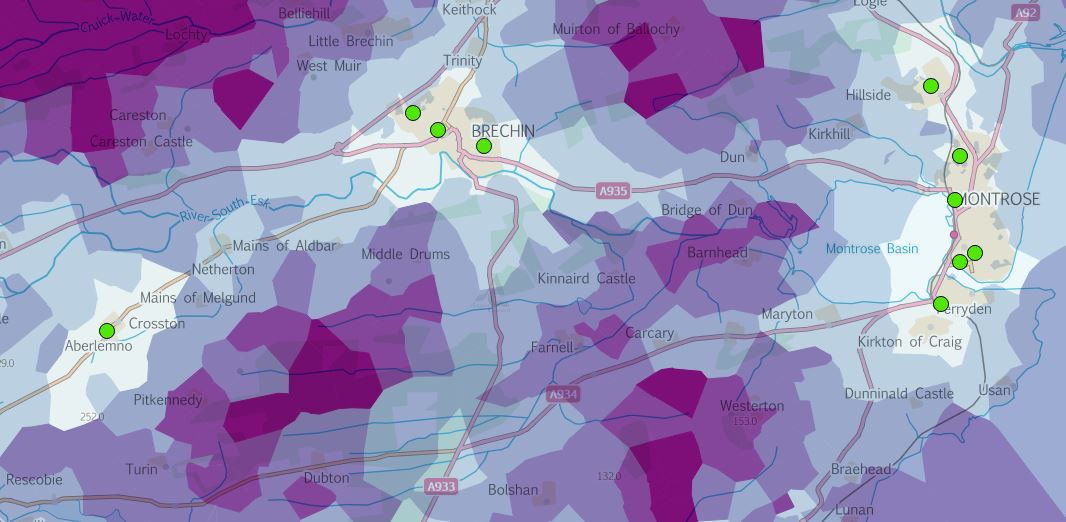# Voronoi isochrones

How can we visualise our driving distance dataset another way? Can we create something like this which is using Voronoi polygons:

Well, yes we can. And PostGIS gives us the tools to put this together very quickly. First, create a view of the distinct nodes in your output table created by my batch function in a previous post.

``````CREATE OR REPLACE VIEW vw_sdd_nodes AS
SELECT DISTINCT ON (node) *
FROM school_driving_distance
ORDER BY node, agg_cost ASC;
``````

(from this StackExchange post).

Then using a new function in PostGIS 2.3.x, ST_VoronoiPolygons, create a voronoi polygon layer based on the road nodes in the view above. See this StackExchange post for more info.

``````CREATE OR REPLACE VIEW vw_sdd_voronoi AS
WITH voronoi AS
(SELECT geometry FROM vw_sdd_nodes)
SELECT
(ST_Dump(ST_SetSRID(ST_CollectionExtract(ST_VoronoiPolygons(ST_Collect(geometry)),3),27700))).geom
AS the_geom
FROM voronoi;
``````

Then, link the nodes back to the voronoi polygons so that each polygon gets a node ID and a cost value in a new table. This takes some time - in my case I had 24,000 nodes intersecting with 24,000 polygons in about 3 minutes.

``````CREATE TABLE edn_sdd_voronoi AS
SELECT row_number() OVER () AS gid, n.node, n.agg_cost, v.the_geom
FROM vw_school_driving_distance_nodes n, vw_sdd_voronoi v
WHERE ST_Intersects(n.geometry, v.the_geom);

CREATE INDEX edn_sdd_voronoi_geometry_ixs
ON corporate.edn_sdd_voronoi
USING gist(the_geom);
``````

I also created a set of Voronoi polygons using the QGIS > Geometry Tools > Voronoi Polygons and that took several hours to create a new shapefile layer. Using PostGIS you can be done in less than 5 minutes depending on how many points you have. As always this could probably be done more efficiently but 4 hours to 5 minutes is good enough for me.

Style the data in QGIS using a graduated renderer and the aggregated cost column and you get something like this.If you have any suggestions for improvements, get in touch.